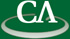### How to find a 2kth root of unity in GF(p) without factoring p–1.

#### 4:00pm, Wednesday June 25th, K9509.

```Abstract

Suppose we want to multiply two polynomials in GF(p)[x] using the Fast
Fourier Transform (FFT).  If n = 2k is the smallest power of 2 greater
than the degree of the product then we can run the FFT in GF(p) if n|(p–1).
To do so we need to find a primitive n'th root of unity ω in GF(p).
Although there are only φ((p–1)/n) such roots, it is easy to find one
if we know a priori a primitive element α in GF(p) as

ω = α(p-1)/n mod p

has order n.  But finding a primitive element requires the factoriation
of p–1 to be known.  Can we efficiently find ω without factoring p–1?
And what should we do if n does not divide p–1?
```